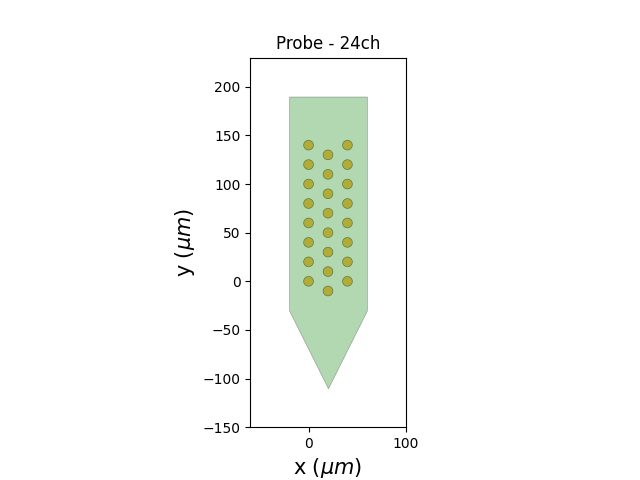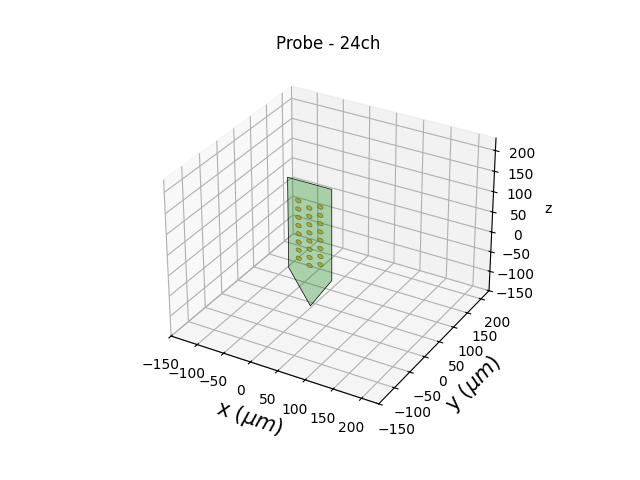# Generate a Probe from scratch¶

This example generates a probe from scratch.

```import numpy as np
import matplotlib.pyplot as plt

from probeinterface import Probe
from probeinterface.plotting import plot_probe
```

First, let’s create dummy positions for a 24-contact probe

```n = 24
positions = np.zeros((n, 2))
for i in range(n):
x = i // 8
y = i % 8
positions[i] = x, y
positions *= 20
positions[8:16, 1] -= 10
```

Now we can create a Probe object  and set the position and shape of each contact

The ndim argument indicates that the contact is 2d, so the positions have a (n_elec, 2) shape.  We can also define a 3d probe with ndim=3 and positions will have a (n_elec, 3) shape.

Note: shapes and shape_params could be arrays as well, indicating the shape for each contact separately.

```probe = Probe(ndim=2, si_units='um')
```

Probe objects have fancy prints!

```print(probe)
```
```Probe - 24ch - 1shanks
```

In addition to contacts, we can create the planar contour (polygon) of the probe

```polygon = [(-20, -30), (20, -110), (60, -30), (60, 190), (-20, 190)]
probe.set_planar_contour(polygon)
```

If pandas is installed, the Probe object can be exported as a dataframe for a simpler view:

```df = probe.to_dataframe()
df
```
x y contact_shapes radius shank_ids contact_ids
0 0.0 0.0 circle 5.0
1 0.0 20.0 circle 5.0
2 0.0 40.0 circle 5.0
3 0.0 60.0 circle 5.0
4 0.0 80.0 circle 5.0
5 0.0 100.0 circle 5.0
6 0.0 120.0 circle 5.0
7 0.0 140.0 circle 5.0
8 20.0 -10.0 circle 5.0
9 20.0 10.0 circle 5.0
10 20.0 30.0 circle 5.0
11 20.0 50.0 circle 5.0
12 20.0 70.0 circle 5.0
13 20.0 90.0 circle 5.0
14 20.0 110.0 circle 5.0
15 20.0 130.0 circle 5.0
16 40.0 0.0 circle 5.0
17 40.0 20.0 circle 5.0
18 40.0 40.0 circle 5.0
19 40.0 60.0 circle 5.0
20 40.0 80.0 circle 5.0
21 40.0 100.0 circle 5.0
22 40.0 120.0 circle 5.0
23 40.0 140.0 circle 5.0

If matplotlib is installed, the Probe can also be easily plotted:

```plot_probe(probe)
``````(<matplotlib.collections.PolyCollection object at 0x7fca48654790>, <matplotlib.collections.PolyCollection object at 0x7fca486aa2f0>)
```

A 2d Probe can be transformed into a 3d Probe by indicating the axes on which contacts will lie (Here the ‘y’ coordinate will be 0 for all contacts):

```probe_3d = probe.to_3d(axes='xz')
plot_probe(probe_3d)

plt.show()
```Total running time of the script: (0 minutes 0.847 seconds)

Gallery generated by Sphinx-Gallery